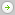What Is the Average Rate of Return?

When you see the word 'average' return, it's worth checking how the average was calculated. Here is an example of two managed funds: Fund A managed by Mr. X, and Fund B managed by Mr. Y.

Mr. X tells potential investors that he has earned Fund A investors an 'average return of 20% per year', compared with Mr. Y whose Fund B earned an average of only 10% per year. Usually you find the average of a set of different values by adding up all the values, and dividing that total by the number of values. This is called the arithmetic mean. Mr. X's Fund A earned 100% in the first year, but then lost 60% in the second year. Using this arithmetic method, we can see that Mr. X can truthfully claim the average of +100% and –60% is 20%.

Mr. Y's Fund B earned just 10% in the first year, and earned 10% again the next year. Of course, Mr. Y's arithmetic average is 10%. An investor who put \$100 into the '20% average' Fund A will see their opening balance of \$100 grow to \$200 at the end of the first year, only to fall to \$80 at the end of the second year. The investor in Mr. Y's Fund B sees a much happier picture. Their opening balance of \$100 grew to \$110 at the end of the first year, and rose again to \$121 after the second year.

Check how the average is worked out

The example shows how the common method for working out averages, called the arithmetic mean, can present a misleading picture to investors. You need a better method to find the average of different rates of return.

One such method is called the geometric mean. Using the geometric mean:

• Mr. X earned his investors –10.56% per year and
• Mr. Y earned 10% per year, a much more useful picture from the investor's point of view.

How to work out average returns on a computer spreadsheet

You can work out the geometric mean by using that function in computer spreadsheet programs, such as Microsoft Excel. Before you start, beware of keying in percentages. Your spreadsheet can find it difficult to work with them. The spreadsheet will refuse to tell you the geometric mean (or give you a wrong answer), if you key in any negative percentages. To solve that problem, first turn your percentages into decimal numbers.

Turn percentage returns into decimal numbers

If you are not sure how to do this, here is an example. If someone asks 'What do you end up with if you invest \$13 and earn 17%?', we find out first that 17% of \$13 equals \$2.21, and then we add that to \$13 to come up with \$15.21.

It would be quicker if we just multiplied \$13 by 1.17. Similarly, if someone asks 'What do you end up with if you invest \$13 but lose 17%?', you could go the long way round or you just could multiply \$13 by 0.83 (1 minus 0.17). Remember a percentage simply divides the number 1 into 100 parts.